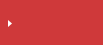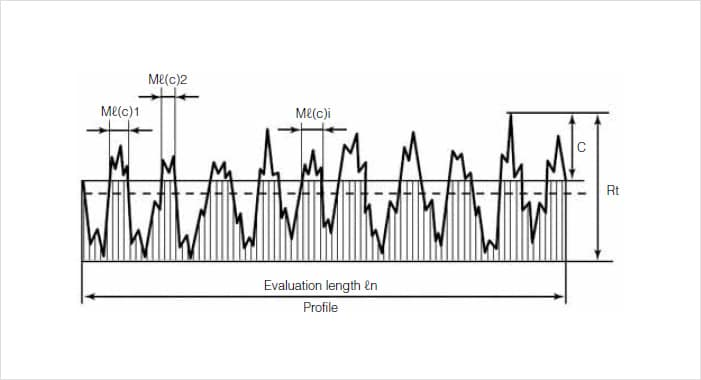>  What is line roughness?  >  Surface Roughness Parameters

# Material Ratio Curve (Bac) | Surface Roughness Parameters

## Surface roughness parameters in JIS B 0601

• Peaks and valleys in the height direction
• Average amplitude in the height direction
• Average characteristics in the height direction
• Horizontal direction
• Hybrid
• Areal material ratio curve and probability density functionSearch from the parameters list

## Material Ratio Curve (Bac)

Material ratio curve shows the curve of load length ratio determined as the cut level c function.

Load length ratio is the ratio (%) obtained by dividing the total lengths of the length of curve data exceeding the cut level by the entire length (rn).The number of data points N is the smaller of 4096 and (maximum height / Z calibration). Maximum height is divided by (N-1) to get the pitch, which is then used for the cut level.
Each height is assigned BAC (n) as its load length ratio. n = N is the minimum height.
BAC (N) = 100.0, however BAC (0) does not necessarily equal 0.0.
Load length ratio is displayed as a % on the horizontal axis while cut level is displayed on the vertical axis in μm Mil. (Cut height is in the same range as the profile curve graph.)

## Surface Roughness Parameters

This section explains the main parameters of ISO 4287:1997. Each parameter is classified according to primary profile (P), roughness profile (R), and waviness profile (W) in order to evaluate different aspects of the profile. (When the wavelengths of the waviness and primary profile components are compared, the surface roughness component is the asperity component of that which has the comparatively shorter wavelength.)## Search from the parameters list

Peaks and valleys in the height direction Arithmetical mean height (Ra, Pa, Wa) Maximum height of profile (Rz, Pz, Wz) Maximum profile peak height (Rp, Pp, Wp) Maximum profile valley depth (Rv, Pv, Wv) Mean height of profile elements (Rc, Pc, Wc) Total height of profile (Rt, Pt, Wt) Root mean square deviation (Rq, Pq, Wq) Skewness (Rsk, Psk, Wsk) Kurtosis (Rku, Pku, Wku) Mean width of the profile elements (RSm, PSm, WSm) Root mean square slope (RΔq, PΔq, WΔq) Load length ratio (Rmr (c), Pmr (c), Wmr (c)) Profile cut level difference (Rδc, Pδc, Wδc) Relative load length ratio (Rmr, Pmr, Wmr) Material ratio curve (BAC) Probability density function (ADF)